Most Affordable JEE | NEET | 8,9,10 Preparation by Kota's Top IITian Doctor Faculties

# NCERT Solutions for Class 11 Physics chapter 3 - Motion in a straight Line - Free PDF Download`
Hey, are you a class 11 student and looking for ways to download NCERT Solutions for Class 11 Physics chapter 3 "Motion in a straight Line"? If yes. Then read this post till the end.

In this article, we have listed NCERT solution for class 11 Physics Chapter 3 in PDF that are prepared by Kota’s top IITian’s Faculties by keeping Simplicity in mind.

If you want to learn and understand class 11 Physics chapter 3 "Motion in a straight Line" in an easy way then you can use these solutions PDF.

NCERT Solutions helps students to Practice important concepts of subjects easily. Class 11 Physics solutions provide detailed explanations of all the NCERT questions that students can use to clear their doubts instantly.

If you want to score high in your class 11 Physics Exam then it is very important for you to have a good knowledge of all the important topics, so to learn and practice those topics you can use eSaral NCERT Solutions.

So, without wasting more time Let’s start.

### Download The PDF of NCERT Solutions for Class 11 Physics Chapter 3 "Motion in a straight Line"

Question 1. In which of the following examples of motion, can the body be considered approximately a point object:
(a) a railway carriage moving without jerks between two stations.
(b) a monkey sitting on top of a man cycling smoothly on a circular track.
(c) a spinning cricket ball that turns sharply on hitting the ground.
(d) a tumbling beaker that has slipped off the edge of a table.

Solution: (a), (b)
(a) The carriage can be treated as a point-sized object as the size of a carriage is very small as compared to the distance between two stations.
(b) The monkey can be considered as a point-sized object on the track because the size of a monkey is very small as compared to the size of a circular track
(c) The cricket ball cannot be considered as a point object as the size of a spinning cricket ball is comparable to the distance through which it turns sharply on hitting the ground.
(d) The beaker cannot be considered as a point object as the size of a beaker is comparable to the height of the table from which it slipped.

Question 2. The position-time (x-t) graphs for two children A and B returning from their school O to their homes P and Q respectively are shown in Figure. Choose the correct entries in the brackets below;
(a) (A/B) lives closer to the school than (B/A)
(b) (A/B) starts from the school earlier than (B/A)
(c) (A/B) walks faster than (B/A)
(d) A and B reach home at the (same/different) time
(e) (A/B) overtakes (B/A) on the road (once/twice).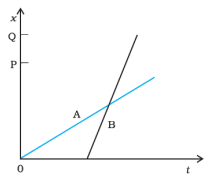Solution: (a) A lives closer to school than B.
(b) A start from school earlier than B.
(c) B walks faster than A.
(d) A and B reach home at the same time.
(e) B overtakes A once on the road.
Explanation:
(a) The distance of the school from the A's home is less than that from B's home because, in the given distance-time graph, it can be observed that distance OP < OQ.
(b) In the given graph, it can be observed that for x = 0,t = 0 for A, whereas for 𝑥 = 0, t has some finite value for B. Thus, A starts his journey from school earlier than B
(c) It can be noted in the given x-t graph that B's slope is greater than A's. Since the x-t graph's slope gives the speed, a greater slope implies B's speed is higher than A's speed.
(d) The graph shows that both A and B reach their respective homes simultaneously.
(e) B moves later than A and its speed is greater than A. It is evident from the graph that B only once overtakes A on the highway.

Question 3. A woman starts from her home at $9.00 \mathrm{am}$, walks with a speed of $5 \mathrm{~km} \mathrm{~h}^{-1}$ on a straight road up to her office $2.5 \mathrm{~km}$ away, stays at the office up to $5.00 \mathrm{pm}$, and returns home by an auto with a speed of $25 \mathrm{~km} \mathrm{~h}^{-1}$. Choose suitable scales and plot the $x-t$ graph of her motion.
Solution: The Speed of the woman is 5 km⁄h.
The Distance between her office and home is 2.5 km
Time = Distance / Speed
$=\frac{2.5}{5}=0.5 \mathrm{~h}=30 \mathrm{~min}$
The woman covers the same distance in the evening by an auto
Now, speed of the auto = 25 km⁄h
Time taken = Distance / Speed
$=\frac{2.5}{25}=\frac{1}{10}=0.1 \mathrm{~h}=6 \mathrm{~min}$
The displacement-time graph of the motion of the woman is shown in the given figure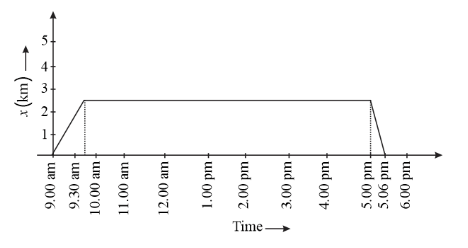Question 4. A drunkard walking in a narrow lane takes 5 steps forward and 3 steps backward, followed again by 5 steps forward and 3 steps backward, and so on. Each step is $1 \mathrm{~m}$ long and requires $1 \mathrm{~s}$. Plot the $x-t$ graph of his motion. Determine graphically and otherwise how long the drunkard takes to fall in a pit $13 \mathrm{~m}$ away from the start.

Solution: Given:
Distance covered with 1 step $=1 \mathrm{~m}$
Time taken $=1 \mathrm{~s}$
Time taken to move first $5 \mathrm{~m}$ forward $=5 \mathrm{~s}$
Time taken to move $3 \mathrm{~m}$ backwards $=3 \mathrm{~s}$
Net distance covered $=5-3=2 \mathrm{~m}$
Net time taken to cover $2 \mathrm{~m}=8 \mathrm{~s}$
Drunkard covers $2 \mathrm{~m}$ in $8 \mathrm{~s}$.
Drunkard covered $4 \mathrm{~m}$ in $16 \mathrm{~s}$.
Drunkard covered $6 \mathrm{~m}$ in $24 \mathrm{~s}$.
Drunkard covered $8 \mathrm{~m}$ in $32 \mathrm{~s}$.
The drunkard will cover a distance of $5 \mathrm{~m}$ and a total distance of $13 \mathrm{~m}$ in the next $5 \mathrm{~s}$ and will fall into the pit.
The drunkard's net time to cover $13 m=32+5=37 \mathrm{~s}$
The displacement-time graph of the drunkard's motion can be shown as: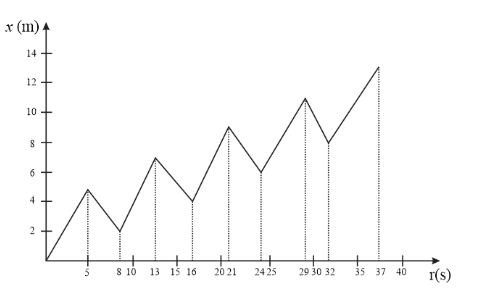Question 5. A jet aeroplane travelling at the speed of $500 \mathrm{~km} \mathrm{~h}^{-1}$ ejects its products of combustion at the speed of $1500 \mathrm{~km} \mathrm{~h}^{-1}$ relative to the jet plane. What is the speed of the latter with respect to an observer on the ground?
Solution:
Given:
Speed of the jet aeroplane, $v_{j e t}=500 \mathrm{~km} / \mathrm{h}$
The Relative speed of its products of combustion with respect to the plane,
$v_{\text {smoke }}=-1500 \mathrm{~km} / \mathrm{h}$
Speed combustion products with respect to the ground $=\mathrm{v}_{\text {smoke }}^{\prime 2}$
The relative speed of its products of combustion with respect to the Aeroplan,
$\mathrm{v}_{\text {smoke }}=\mathrm{v}_{\text {smoke }}^{\prime 2}-\mathrm{V}_{\text {jet }}$
$-1500=v_{\text {smoke }}^{\prime 2}-500$
$v_{\text {smoke }}^{\prime 2}=-1000 \mathrm{~km} / \mathrm{h}$
The negative sign indicates that the direction of its products of combustion is opposite to the direction of motion of the jet Aeroplan.

Question 6. A car moving along a straight highway with a speed of $126 \mathrm{~km} \mathrm{~h}^{-1}$ is brought to a stop within a distance of $200 \mathrm{~m}$. What is the retardation of the car (assumed uniform), and how long does it take for the car to stop?

Solution:
Initial velocity of the car, $\mathrm{u}=126 \mathrm{~km} / \mathrm{h}=35 \mathrm{~m} / \mathrm{s}$
The final velocity of the car, $\mathrm{v}=0$
The distance covered by the car before the rest, $\mathrm{s}=200 \mathrm{~m}$
Retardation produced in the car $=\mathrm{a}$
From the third equation of motion, a can be calculated as:
$\mathrm{v}^{2}-\mathrm{u}^{2}=2 \mathrm{as}$
$(0)^{2}-(35)^{2}=2 \times \mathrm{a} \times 200$
$\mathrm{a}=-\frac{(35 \times 35)}{(2 \times 200)}$
$\mathrm{a}=-3.06 \mathrm{~m} / \mathrm{s}^{2}$
From the first equation of motion, time (t) taken by the car to stop can be obtained as:
$\mathrm{v}=\mathrm{u}+\mathrm{at}$
$\mathrm{t}=\frac{\mathrm{v}-\mathrm{u}}{\mathrm{a}}=-\frac{35}{-3.06}=11.44 \mathrm{~s}$

Question 7. Two trains $\mathrm{A}$ and $\mathrm{B}$ of length $400 \mathrm{~m}$ each are moving on two parallel tracks with a uniform speed of $72 \mathrm{~km} \mathrm{~h}^{-1}$ in the same direction, with $\mathrm{A}$ ahead of $\mathrm{B}$. The driver of $\mathrm{B}$ decides to overtake $\mathrm{A}$ and accelerates by $1 \mathrm{~m} / \mathrm{s}^{2}$. If after $50 \mathrm{~s}$, the guard of $\mathrm{B}$ just brushes past the driver of A, what was the original distance between them?

Solution:
For train A:
Initial velocity, $\mathrm{u}=72 \frac{\mathrm{km}}{\mathrm{h}}=20 \frac{\mathrm{m}}{\mathrm{s}}$
Time, $\mathrm{t}=50 \mathrm{~s}$
Acceleration, $a_{1}=0$ (Since it is moving with a uniform velocity)
From the second equation of motion, the distance $\left(\mathrm{s}_{\mathrm{I}}\right)$ covered by train $\mathrm{A}$ can be obtained as:
$s_{I}=u t+\frac{1}{2} a_{I} t^{2}$
$=20 \times 50+0=1000 \mathrm{~m}$
For train B:
Initial velocity, $\mathrm{u}=72 \mathrm{~km} / \mathrm{h}=20 \mathrm{~m} / \mathrm{s}$
Acceleration, $\mathrm{a}=1 \mathrm{~m} / \mathrm{s}^{2}$
Time, $\mathrm{t}=50 \mathrm{~s}$
From the second equation of motion, distance $\left(\mathrm{s}_{\mathrm{II}}\right)$ covered by train $\mathrm{A}$ can be obtained as:
$s_{I I}=u t+\frac{1}{2} a t^{2}$
$=20 \times 50 \times \frac{1}{2} \times 1 \times(50)^{2}=2250 \mathrm{~m}$
Hence, The original distance between the A train driver and the B train guard
$=2250-1000=1250 \mathrm{~m}$

Question 8. On a two-lane road, car $A$ is travelling with a speed of $36 \mathrm{~km} \mathrm{~h}^{-1}$. Two cars $B$ and $C$ approach car $\mathrm{A}$ in opposite directions with a speed of $54 \mathrm{~km} \mathrm{~h}^{-1}$ each. At a certain instant, when the distance $\mathrm{AB}$ is equal to $\mathrm{AC}$, both being $1 \mathrm{~km}, \mathrm{~B}$ decides to overtake $A$ before $C$ does. What minimum acceleration of car B is required to avoid an accident?

Solution: The velocity of $\operatorname{car} A, v_{x}=36 \mathrm{~km} / \mathrm{h}=10 \mathrm{~m} / \mathrm{s}$
The velocity of $\operatorname{car} A, v_{y}=54 \mathrm{~km} / \mathrm{h}=15 \mathrm{~m} / \mathrm{s}$
The velocity of car $C, v_{z}=54 \mathrm{~km} / \mathrm{h}=15 \mathrm{~m} / \mathrm{s}$
The Relative velocity of car $B$ with respect to $\operatorname{car} A$,
$v_{y x}=v_{y}-v_{x}=15-10=5 \mathrm{~m} / \mathrm{s}$
\ The relative velocity of $\operatorname{car} \mathrm{C}$ with respect to car $\mathrm{A}$,
$v_{z x}=v_{z}-\left(-v_{x}\right)=15+10=25 \mathrm{~m} / \mathrm{s}$
At a certain distance, both cars $\mathrm{B}$ and $\mathrm{C}$ are at the same distance from $\mathrm{car} A$ i.e.,
$s=1 \mathrm{~km}=1000 \mathrm{~m}$
The Time is taken $(t)$ by $\operatorname{car} C$ to cover $1000 \mathrm{~m}=\frac{1000}{25}=40 \mathrm{~s}$
Hence, to avoid an accident, car B must cover the same distance in a maximum of $40 \mathrm{~s}$. From the second equation of motion, minimum acceleration (a) produced by car B can be obtained as:
$s=u t+\frac{1}{2} a t^{2}$
$1000=5 \times 40+\frac{1}{2} \times \mathrm{a} \times 40^{2}$
$\mathrm{a}=\frac{1600}{1600}=1 \mathrm{~m} / \mathrm{s}^{2}$

Question 9. Two towns $\mathrm{A}$ and $\mathrm{B}$ are connected by regular bus service with a bus leaving in either direction every T minutes. A man cycling with a speed of $20 \mathrm{~km} \mathrm{~h}^{-1}$ in the direction, $\mathrm{A}$ to $\mathrm{B}$ notices that a bus goes past him every $18 \mathrm{~min}$ in the direction of his motion, and every 6 min in the opposite direction. What is the period $T$ of the bus service and with what speed (assumed constant) do the buses ply on the road?

Solution: Let $V$ be the speed of the bus running between towns $A$ and $B$.
Speed of the cyclist, $v=20 \mathrm{~km} / \mathrm{h}$
The relative speed of the bus moving in the direction of the cyclist
$=V-v=(V-20) \mathrm{km} / \mathrm{h}$
The bus went past the cyclist every 18 min i.e. $18 / 60 \mathrm{~h}$, (when he moves in the direction of the bus).
Distance covered by the bus $=(V-20) \frac{18}{60} \ldots \ldots \ldots \ldots . .$ (i)
Since one bus leaves after every T minutes, the distance travelled by bus will be equal to: $V \times \frac{T}{60} \ldots \ldots \ldots \ldots$ (ii)
Both equations (i) $\quad$ and (ii) are equal.
$(V-20) \times \frac{18}{60}=\frac{V T}{60} \ldots \ldots \ldots \ldots$ (iii)
The relative speed of the bus moving in the opposite direction of the cyclist = $(V+20) \mathrm{km} / \mathrm{h}$
Time taken by the bus to go past the cyclist $=6 \min =\frac{6}{60} \mathrm{~h}$
$\therefore(V+20) \times \frac{6}{60}=\frac{V T}{60} \ldots \ldots \ldots \ldots . .$ (iv)
From equations (iii) and (iv), we get
$(V+20) \times \frac{6}{60}=(\mathrm{V}-20) \times \frac{18}{60}$
$V+20=3 \mathrm{~V}-60$
$2 \mathrm{~V}=80$
$V=40 \mathrm{~km} / \mathrm{h}$
Substituting the value of $V$ in equation (iv), we get
$(40+20) \times \frac{6}{60}=\frac{40 T}{60}$
$60 \times \frac{6}{60}=\frac{40 T}{60}$
$6=\frac{40 T}{60}$
$\Rightarrow 40 \mathrm{~T}=6 \times 60$
$\Rightarrow 40 \mathrm{~T}=360$
$\Rightarrow \mathrm{T}=\frac{360}{40}$
$\Rightarrow \mathrm{T}=9 \mathrm{~min}$

Question 10. A player throws a ball upwards with an initial speed of $29.4 \mathrm{~m} \mathrm{~s}^{-1}$.
(a) What is the the direction of acceleration during the upward motion of the ball?
(b) What are the velocity and acceleration of the ball at the highest point of its motion?
(c) Choose the $\mathrm{x}=0 \mathrm{~m}$ and $\mathrm{t}=0 \mathrm{~s}$ to be the location and time of the ball at its highest point, vertically downward direction to be the positive direction of the $x$ -axis, and give the signs of position, velocity and acceleration of the ball during its upward, and downward motion.
(d) To what height does the ball rise and after how long does the ball return to the player's hands? (Take $g=9.8 \mathrm{~m} \mathrm{~s}^{-2}$ and neglect air resistance).

Solution: (a) Irrespective of the direction of the motion of the ball, acceleration (which is actually acceleration due to gravity) always acts in the downward direction towards the centre of the Earth.

(b) At maximum height, the velocity of the ball becomes zero. Acceleration due to gravity at a given place is constant and acts on the ball at all points (including the highest point) with a constant value, i.e., $9.8 \mathrm{~m} / \mathrm{s}^{2}$.
(c) During upward motion, the sign of position is positive, the sign of velocity is negative, and a sign of acceleration is positive. During downward motion, the signs of position, velocity, and acceleration are all positive.
(d) The initial velocity of the ball, $\mathrm{u}=29.4 \mathrm{~m} / \mathrm{s}$
The final velocity of the ball, $\mathrm{v}=0$ (At maximum height, the velocity of the ball becomes zero)
Acceleration, $\mathrm{a}=-g=-9.8 \mathrm{~m} / \mathrm{s}^{2}$
From the third equation of motion, height (s) can be calculated as:
$\mathrm{v}^{2}-\mathrm{u}^{2}=2 g \mathrm{~s}$
$s=\frac{v^{2}-u^{2}}{2 g}$
$=\frac{(0)^{2}-(29.4)^{2}}{2 \times(-9.8)}=44.1 \mathrm{~m}$
$=\frac{(0)^{2}-(29.4)^{2}}{2 \times(-9.8)}=44.1 \mathrm{~m}$
From the first equation of motion, time of ascent $(\mathrm{t})$ is given as:
$\mathrm{v}=\mathrm{u}+\mathrm{at}$
$\mathrm{t}=\frac{\mathrm{v}-\mathrm{u}}{\mathrm{a}}=-\frac{29.4}{-9.8}=3 \mathrm{~s}$
Time of ascent $=$ Time of descent
Hence, the total time taken by the ball to return to the player's hands $=3+3=$ $6 \mathrm{~s}$

Question 11. Read each statement below carefully and state with reasons and examples if it is true or false; A particle in one-dimensional motion
(a) with zero speed at an instant may have non-zero acceleration at that instant
(b) with zero speed may have non-zero velocity,
(c) with constant speed must have zero acceleration,
(d) with a positive value of acceleration must be speeding up.

Solution:
(a) True. When an object is thrown vertically up in the air, its speed becomes zero at maximum height. However, it has an acceleration equal to the acceleration due to gravity (g) that acts in the downward direction at that point.
(b) False. Speed is the magnitude of velocity. When speed is zero, the magnitude of velocity along with the velocity is zero.
(c) True. A car moving on a straight highway with constant speed will have a constant velocity. Since acceleration is defined as the rate of change of velocity, acceleration of the car is also zero.
(d) False. This statement is false in the situation when acceleration is positive, and velocity is negative at the instant time taken as origin. Then, for all the time before velocity becomes zero, there is slowing down of the particle. Such a case happens when a particle is projected upwards.
This statement is true when both velocity and acceleration are positive, at the instant time taken as origin. Such a case happens when a particle is moving with positive acceleration or falling vertically downwards from a height.

Question 12. A ball is dropped from a height of $90 \mathrm{~m}$ on a floor. At each collision with the floor, the ball loses one-tenth of its speed. Plot the speed-time graph of its motion between $\mathrm{t}=0$ to $12 \mathrm{~s}$

Solution:
The ball is dropped from a height, $s=90 \mathrm{~m}$
The initial velocity of the ball, $\mathrm{u}=0$
Acceleration, $a=g=9.8 \mathrm{~m} / \mathrm{s}^{2}$
The final velocity of the ball $=v$
From the second equation of motion, time (t) taken by the ball to hit the ground can be obtained as:
$s=u t+\frac{1}{2} a t^{2}$
$90=0+\frac{1}{2} \times 9.8 \times t^{2}$
$t=\sqrt{18.38}=4.29 \mathrm{~s}$
From the first equation of motion, the final velocity is given as:
$v=u+a t$
$=0+9.8 \times 4.29=42.04 \mathrm{~m} / \mathrm{s}$
Rebound velocity of the ball, $u_{r}=\frac{9}{10} v=\frac{9}{10} \times 42.04=37.84 \mathrm{~m} / \mathrm{s}$
Time (t) taken by the ball to reach maximum height is obtained with the help of the first equation of motion as:
$\mathrm{v}=\mathrm{ur}+\mathrm{at}^{\prime}$
$0=37.84+(-9.8) \mathrm{t}^{\prime}$
$\mathrm{t}^{\prime}=\frac{-37.84}{-9.8}=3.86 \mathrm{~s}$
Total time is taken by the ball $=\mathrm{t}+\mathrm{t}^{\prime}=4.29+3.86=8.15 \mathrm{~s}$
As the time of ascent is equal to the time of descent, the ball takes $3.86 \mathrm{~s}$ to strike back on the floor for the second time.
The velocity with which the ball rebounds from the floor $=\frac{9}{10} \times 37.84=$ $34.05 \mathrm{~m} / \mathrm{s}$
Total time taken by the ball for the second rebound $=8.15+3.86=12.01 \mathrm{~s}$
The speed-time graph of the ball is represented in the given figure as: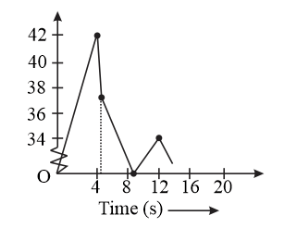Question 13. Explain clearly, with examples, the distinction between:
(a) magnitude of displacement (sometimes called distance) over an interval of time, and the total length of path covered by a particle over the same interval;
(b) magnitude of average velocity over an interval of time, and the average speed over the same interval. [Average speed of a particle over an interval of time is defined as the total path length divided by the time interval]. Show in both (a) and (b) that the second quantity is either greater than or equal to the first.
When is the equality sign true? [For simplicity, consider one-dimensional motion only].

Solution:
(a) The magnitude of displacement over an interval of time is the shortest distance (which is a straight line) between the initial and final positions of the particle. The total path length of a particle is the actual path length covered by the particle in a given interval of time.
For example, suppose a particle moves from point $\mathrm{A}$ to point $\mathrm{B}$ and then, comes back to a point, $\mathrm{C}$ taking a total time $\mathrm{t}$, as shown below then, the magnitude of displacement of the particle $=A C$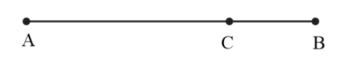Whereas, total path length $=\mathrm{AB}+\mathrm{BC}$ It is also important to note that the magnitude of displacement can never be greater than the total path length. However, in some cases, both quantities are equal to each other
(b) The magnitude of average velocity = Magnitude of displacement / Time Interval For the given particle,
Average velocity $=\frac{\mathrm{AC}}{\mathrm{t}}$
Average Speed $=\frac{\text { Total path lenght }}{\text { Time interval }}$
$==\frac{A B+B C}{t}$
Since $(\mathrm{AB}+\mathrm{BC})>\mathrm{AC}$, average speed is greater than the magnitude of average velocity. The two quantities will be equal if the particle continues to move along a straight line.

Question 14. A man walks on a straight road from his home to a market $2.5 \mathrm{~km}$ away with a speed of $5 \mathrm{~km} \mathrm{~h}^{-1}$. Finding the market closed, he instantly turns and walks back home with a speed of $7.5 \mathrm{~km} \mathrm{~h}^{-1}$. What is the
(a) magnitude of average velocity, and
(b) average speed of the man over the interval of time (i) 0 to $30 \mathrm{~min}$, (ii) 0 to $50 \mathrm{~min}$, (iii) 0 to $40 \mathrm{~min}$ ?
[Note: You will appreciate from this exercise why it is better to define average speed as total path length divided by time, and not as the magnitude of average velocity. You would not like to tell the tired man on his return home that his average speed was zero!]

Solution:
(i) Time is taken by the man to reach the market from home, $t_{1}=\frac{2.5}{5}=\frac{1}{2} h=$ $30 \mathrm{~min}$
Time taken by the man to reach home from the market, $t_{2}=\frac{2.5}{7.5}=\frac{1}{3} h=$ $20 \mathrm{~min}$
Total time taken in the whole journey $=30+20=50 \mathrm{~min}$
(a) $\quad$ Average velocity $=\frac{\text { Displacement }}{\text { Time }}=\frac{2.5}{\frac{1}{2}}=5 \mathrm{~km} / \mathrm{h}$
(b) Average speed $=\frac{\text { Distance }}{\text { Time }}=\frac{2.5}{\frac{1}{2}}=5 \mathrm{~km} / \mathrm{h}$
(ii) $\quad$ Time $=50 \mathrm{~min}=\frac{5}{6} \mathrm{~h}$
Net displacement $=0$
Total distance $=2.5+2.5=5 \mathrm{~km}$
(a) Average velocity $=\frac{\text { Displacement }}{\text { Time }}=0$
(b) Average speed $=\frac{\text { Distance }}{\text { Time }}=\frac{5}{\frac{5}{6}}=6 \mathrm{~km} / \mathrm{h}$
(iii) $\quad$ Speed of the $\operatorname{man}=7.5 \mathrm{~km}$
Distance travelled in first $30 \min =2.5 \mathrm{~km}$
Distance travelled by the man (from market to home) in the next $10 \mathrm{~min}$
$=7.5 \times \frac{10}{60}=1.25 \mathrm{~km}$
Net displacement $=2.5-1.25=1.25 \mathrm{~km}$
Total distance travelled $=2.5+1.25=3.75 \mathrm{~km}$
(a) Average Velocity $=\frac{1.25}{\left(\frac{40}{60}\right)}=1.25 \times \frac{3}{2}=1.875 \mathrm{~km} / \mathrm{h}$
(b) Average Speed $=\frac{3.75}{\left(\frac{40}{60}\right)}=5.625 \mathrm{~km} / \mathrm{h}$’

Question 15. In Exercises $3.13$ and 3.14, we have carefully distinguished between average speed and magnitude of average velocity. No such distinction is necessary when we consider instantaneous speed and magnitude of velocity. The instantaneous speed is always equal to the magnitude of instantaneous velocity. Why?

Solution: Instantaneous velocity is given by the first derivative of distance with respect to time i.e.,
$\mathrm{V}_{\mathrm{ln}}=\frac{\mathrm{dx}}{\mathrm{dt}}$
Here, the time interval dt is so small that it is assumed that the particle does not change its direction of motion. As a result, both the total path length and magnitude of displacement become equal is this interval of time.

Question 16. Look at the graphs (a) to (d) (Fig. 3.20) carefully and state, with reasons, which of these cannot possibly represent one-dimensional motion of a particle.
(a)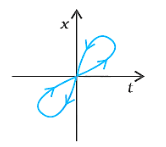(b)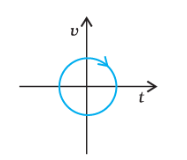(c)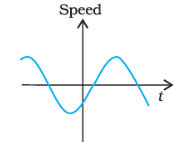(d)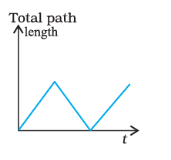Solution:
(a) The given $\mathrm{x}-\mathrm{t}$ graph, shown in (a), does not represent one-dimensional motion of the particle. This is because a particle cannot have two positions at the same instant of time.
(b) The given $\mathrm{v}-\mathrm{t}$ graph, shown in (c), does not represent one-dimensional motion of the particle. This is because a particle can never have two values of velocity at the same instant of time.
(c) The given $\mathrm{v}-\mathrm{t}$ graph, shown in (c), does not represent one-dimensional motion of the particle. This is because speed being a scalar quantity cannot be negative. (d) The given $v-t$ graph, shown in (d), does not represent one-dimensional motion of the particle. This is because the total path length travelled by the particle cannot decrease with time.

Question 17. Figure shows the $x-t$ plot of one-dimensional motion of a particle. Is it correct to say from the graph that the particle moves in a straight line for $t<0$ and on a parabolic path for $t>0$ ? If not, suggest a suitable physical context for this graph.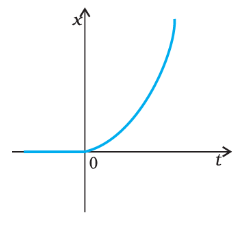Solution:
No
The $x-t$ graph of a particle moving in a straight line for $t<0$ and on a parabolic path for $t>0$ cannot be shown as the given graph. This is because, the given particle does not follow the trajectory of path followed by the particle as $t=0, x=$0. A physical situation that resembles the above graph is of a freely falling body held for some time at a height.

Question 18. A police van moving on a highway with a speed of $30 \mathrm{~km} \mathrm{~h}^{-1}$ fires a bullet at a thief's car speeding away in the same direction with a speed of $192 \mathrm{~km} \mathrm{~h}^{-1}$. If the muzzle speed of the bullet is $150 \mathrm{~m} \mathrm{~s}^{-1}$, with what speed does the bullet hit the thief's car?
(Note: Obtain that speed which is relevant for damaging the thief's car).
Solution:
Speed of the police van, $v_{p}=30 \mathrm{~km} / \mathrm{h}=8.33 \mathrm{~m} / \mathrm{s}$
Muzzle speed of the bullet, $v_{b}=150 \mathrm{~m} / \mathrm{s}$
Speed of the thief's car, $v_{t}=192 \mathrm{~km} / \mathrm{h}=53.33 \mathrm{~m} / \mathrm{s}$
Since the bullet is fired from a moving van, its resultant speed can be obtained as:
$=150+8.33=158.33 \mathrm{~m} / \mathrm{s}$
Since both the vehicles are moving in the same direction, the velocity with which the bullet hits the thief's car can be obtained as:
$v_{b t}=v b-v t$
$=158.33-53.33=105 \mathrm{~m} / \mathrm{s}$

3.19. Suggest a suitable physical situation for each of the following graph’s figure:
(a)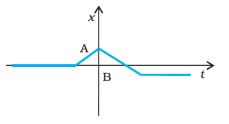(b)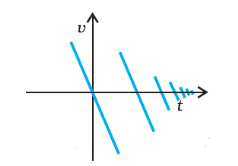(c)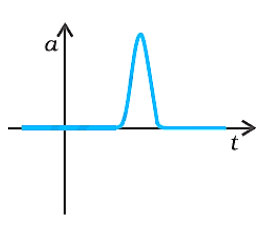Solution:
(a) The given $\mathrm{x}$ -t graph shows that initially a body was at rest. Then, its velocity increases with time and attains an instantaneous constant value. The velocity then reduces to zero with an increase in time. Then, its velocity increases with time in the opposite direction and acquires a constant value. A similar physical situation arises when a football (initially kept at rest) is kicked and gets rebound from a rigid wall so that its speed gets reduced. Then, it passes from the player who has kicked it and ultimately gets stopped after sometime.
(b) In the given $v$ -tgraph, the sign of velocity changes and its magnitude decreases with a passage of time. A similar situation arises when a ball is dropped on the hard floor from a height. It strikes the floor with some velocity and upon rebound, its velocity decreases by a factor. This continues till the velocity of the ball eventually becomes zero.
(c) The given a-t graph reveals that initially the body is moving with a certain uniform velocity. Its acceleration increases for a short interval of time, which again drops to zero. This indicates that the body again starts moving with the same constant velocity. A similar physical situation arises when a hammer moving with a uniform velocity strikes a nail.

Question 20. Figure given shows the $x-t$ plot of a particle executing one-dimensional simple harmonic motion. (You will learn about this motion in more detail in Chapter14). Give the signs of position, velocity and acceleration variables of the particle at $t=$ $0.3 \mathrm{~s}, 1.2 \mathrm{~s},-1.2 \mathrm{~s}$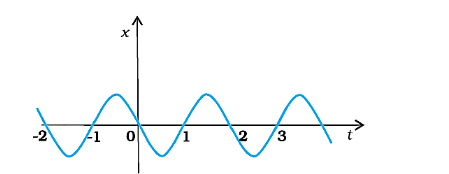Solution:
Negative, Negative, Positive (at $t=0.3 \mathrm{~s}$ )
Positive, Positive, Negative (at $t=1.2 \mathrm{~s}$ )
Negative, Positive, Positive (at $t=-1.2 \mathrm{~s}$ )
For simple harmonic motion (SHM) of a particle, acceleration (a) is given by the relation:
$a=-\omega^{2} x$, where $\omega$ is angular frequency $\ldots \ldots \ldots \ldots \ldots$ (i)
$\mathrm{t}=0.3 \mathrm{~s}$
In this time interval, $\mathrm{x}$ is negative. Thus, the slope of the $\mathrm{x}-\mathrm{t}$ plot will also be negative.
Therefore, both position and velocity are negative.
However, using equation (i), acceleration of the particle will be positive.
$t=1.2 \mathrm{~s}$
In this time interval, $x$ is positive.
Thus, the slope of the $x$ -t plot will also be positive.
Therefore, both position and velocity are positive.
However, using equation (i), acceleration of the particle comes to be negative.
$t=-1.2 \mathrm{~s}$
In this time interval, $x$ is negative. Thus, the slope of the $x-t$ plot will also be negative. Since both $\mathrm{x}$ and $\mathrm{t}$ are negative, the velocity comes to be positive. From equation (i), it can be inferred that the acceleration of the particle will be positive.

Question 21. Figure given shows the $x-t$ plot of a particle in one-dimensional motion. Three different equal intervals of time are shown. In which interval is the average speed greatest, and in which is it the least? Give the sign of average velocity for each interval.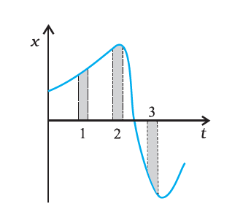Solution:
Interval 3 (Greatest), Interval 2 (Least)
Positive (Intervals $1 \& 2$ ), Negative (Interval 3)
The average speed of a particle shown in the $x-t$ graph is obtained from the slope of the graph in a particular interval of time.
It is clear from the graph that the slope is maximum and minimum restively in intervals 3 and 2 respectively. Therefore, the average speed of the particle is the greatest in interval 3 and is the least in interval $2 .$ The sign of average velocity is positive in both intervals 1 and 2 as the slope is positive in these intervals. However, it is negative in interval 3 because the slope is negative in this interval.
Question 22. Figure gives a speed-time graph of a particle in motion along a constant direction. Three equal intervals of time are shown. In which interval is the average acceleration greatest in magnitude? In which interval is the average speed greatest? Choosing the positive direction as the constant direction of motion, give the signs of $\mathrm{v}$ and a in the three intervals. What are the accelerations at the points $\mathrm{A}, \mathrm{B}, \mathrm{C}$ and $\mathrm{D}$?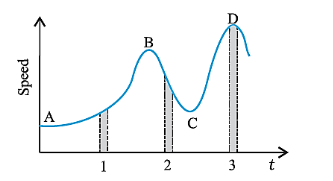Solution:
Average acceleration is greatest in interval 2
Average speed is greatest in interval 3
$\mathrm{v}$ is positive in intervals 1,2, and 3
a is positive in intervals 1 and 3 and negative in interval 2
$a=0$ at $A, B, C, D$
Acceleration is given by the slope of the speed-time graph. In the given case, it is given by the slope of the speed-time graph within the given interval of time.
Since the slope of the given speed-time graph is maximum in interval 2 , average acceleration will be the greatest in this interval.
Height of the curve from the time-axis gives the average speed of the particle. It is clear that the height is the greatest in interval 3. Hence, average speed of the particle is the greatest in interval 3.
In interval 1:
The slope of the speed-time graph is positive. Hence, acceleration is positive. Similarly, the speed of the particle is positive in this interval.
In interval 2:
The slope of the speed-time graph is negative. Hence, acceleration is negative in this interval. However, speed is positive because it is a scalar quantity.
In interval 3:
The slope of the speed-time graph is zero. Hence, acceleration is zero in this interval. However, here the particle acquires some uniform speed. It is positive in this interval.
Points A, B, C, and D are all parallel to the time-axis. Hence, the slope is zero at these points. Therefore, at points $\mathrm{A}, \mathrm{B}, \mathrm{C}$, and $\mathrm{D}$, acceleration of the particle is zero.

Question 23. A three-wheeler starts from rest, accelerates uniformly with $1 \mathrm{~m} \mathrm{~s}^{-2}$ on a straight road for $10 \mathrm{~s}$, and then moves with uniform velocity. Plot the distance covered by the vehicle during the nth second $(\mathrm{n}=1,2,3 \ldots .)$ versus $\mathrm{n}$. What do you expect this plot to be during accelerated motion: a straight line or a parabola?

Solution:
$D_{n}=u+\frac{a}{2}(2 n-1) \ldots \ldots \ldots \ldots \ldots . .$ (1)
$u=$ Initial velocity
$n=$ Time $=1,2,3, \ldots \ldots, n$
In the given case,
$u=0$ and $a=1 \mathrm{~m} / \mathrm{s}^{2}$
$\therefore D n=\frac{1}{2}(2 \mathrm{n}-1) \ldots \ldots \ldots \ldots$ (2)
This relation shows that:
$D n \propto n \ldots \ldots$ (iii)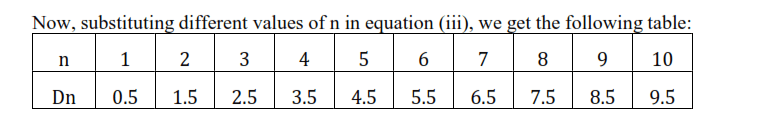The plot between $\mathrm{n}$ and Dn will be a straight line as shown: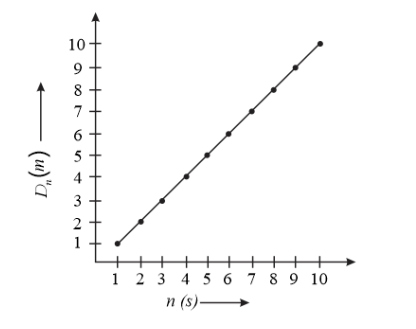Since the given three-wheeler acquires uniform velocity after $10 \mathrm{~s}$, the line will be parallel to the time-axis after $\mathrm{n}=10 \mathrm{~s}$

Question 24. A boy standing on a stationary lift (open from above) throws a ball upwards with the maximum initial speed he can, equal to $49 \mathrm{~m} / \mathrm{s}$. How much time does the ball take to return to his hands? If the lift starts moving up with a uniform speed of $5 \mathrm{~m} / \mathrm{s}$ and the boy again throws the ball up with the maximum speed he can, how long does the ball take to return to his hands?

Solution:
Initial velocity of the ball, $u=49 \mathrm{~m} / \mathrm{s}$
Acceleration, $a=-g=-9.8 \mathrm{~m} / \mathrm{s}^{2}$
Case I:
When the lift was stationary, the boy throws the ball.
Taking upward motion of the ball,
Final velocity, $\mathrm{v}$ of the ball becomes zero at the highest point.
From the first equation of motion, time of ascent (t) is given as:
$v=u+a t$
$t=v-\frac{u}{a}$
$=-\frac{49}{-9.8}=5 \mathrm{~s}$
But, the time of ascent is equal to the time of descent.
Hence, the total time taken by the ball to return to the boy's hand $=5+5=10 \mathrm{~s}$.
Case II:
The lift was moving up with a uniform velocity of $5 \mathrm{~m} / \mathrm{s}$. In this case, the relative velocity of the ball with respect to the boy remains the same i.e., $49 \mathrm{~m} / \mathrm{s}$. Therefore, in this case also, the ball will return back to the boy's hand after $10 \mathrm{~s}$.
Question 25. On a long horizontally moving belt (Figure), a child runs to and fro with a speed $9 \mathrm{~km} \mathrm{~h}^{-1}$ (with respect to the belt) between his father and mother located $50 \mathrm{~m}$ apart on the moving belt. The belt moves with a speed of $4 \mathrm{~km} \mathrm{~h}^{-1}$. For an observer on a stationary platform outside, what is the
(a) speed of the child running in the direction of motion of the belt?
(b) speed of the child running opposite to the direction of motion of the belt?
(c) time taken by the child in (a) and (b)?
Which of the answers alter if motion is viewed by one of the parents?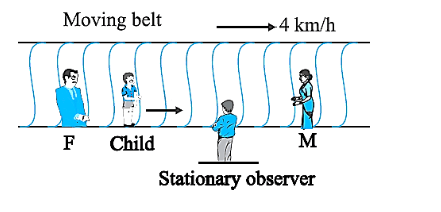Solution:
(a) Speed of the belt, $\mathrm{v}_{\mathrm{B}}=4 \mathrm{~km} / \mathrm{h}$
Speed of the boy, $v_{b}=9 \mathrm{~km} / \mathrm{h}$
Since the boy is running in the same direction of the motion of the belt, his speed
(as observed by the stationary observer) can be obtained as:
$v_{b B}=v_{b}+v_{B}=9+4=13 \mathrm{~km} / \mathrm{h}$
(b) Since the boy is running in the direction opposite to the direction of the motion of the belt, his speed (as observed by the stationary observer) can be obtained as:
$v_{b B}=v_{b}+\left(-v_{B}\right)=9-4=5 \mathrm{~km} / \mathrm{h}$
(c) Distance between the child's parents $=50 \mathrm{~m}$
As both parents are standing on the moving belt, the speed of the child in either direction as observed by the parents will remain the same i.e., $9 \mathrm{~km} / \mathrm{h}=2.5 \mathrm{~m} / \mathrm{s}$
Hence, the time taken by the child to move towards one of his parents is $\frac{50}{2.5}=20 \mathrm{~s}$
If the motion is viewed by any one of the parents, Solution:s obtained in (a) and (b) get altered. This is because the child and his parents are standing on the same belt and hence, are equally affected by the motion of the belt. Therefore, for both parents (irrespective of the direction of motion) the speed of the child remains the same i.e., $9 \mathrm{~km} / \mathrm{h}$
For this reason, it can be concluded that the time taken by the child to reach any one of his parents remains unaltered.
Question 26. Two stones are thrown up simultaneously from the edge of a cliff $200 \mathrm{~m}$ high with initial speeds of $15 \mathrm{~m} \mathrm{~s}^{-1}$ and $30 \mathrm{~m} \mathrm{~s}^{-1}$. Verify that the graph shown in Figure correctly represents the time variation of the relative position of the second stone with respect to the first. Neglect air resistance and assume that the stones do not rebound after hitting the ground. Take $g=10 \mathrm{~m} \mathrm{~s}^{-2}$. Give the equations for the linear and curved parts of the plot.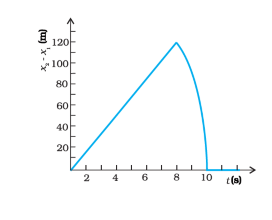Solution:
For first stone:
Initial velocity, $u_{1}=15 \mathrm{~m} / \mathrm{s}$
Acceleration, $a=-g=-10 \mathrm{~m} / \mathrm{s}^{2}$
Using the relation,
$x_{1}=x_{0}+u_{1} t+\frac{1}{2} a t^{2}$
Where, the height of the cliff, $x_{0}=200 \mathrm{~m}$
$x_{1}=200+15 t-5 t^{2} \ldots \ldots \ldots \ldots$ (i)
When this stone hits the ground, $x_{1}=0$
$\therefore-5 t^{2}+15 t+200=0$
$t^{2}-3 t-40=0$
$t^{2}-8 t+5 t-40=0$
$t(t-8)+5(t-8)=0$
$t=8 \mathrm{~s}$ or $t=-5 \mathrm{~s}$
Since the stone was projected at time $t=0$, the negative sign before time is meaningless.
$\therefore t=8 \mathrm{~s}$
For second stone:
Initial velocity, $u_{I I}=30 \mathrm{~m} / \mathrm{s}$
Acceleration, $a=-g=-10 \mathrm{~m} / \mathrm{s}^{2}$
Using the relation,
$x_{2}=x_{0}+u_{l l} t+\frac{1}{2} a t^{2}$
$=200+30 t-5 t^{2} \ldots \ldots \ldots$ (ii)
At the moment when this stone hits the ground; $\mathrm{x}^{2}=0$
$-5 t^{2}+30 t+200=0$
$t^{2}-6 t-40=0$
$t^{2}-10 t+4 t+40=0$
$t(t-10)+4(t-10)=0$
$t(t-10)(t+4)=0 t$
$=10 s$ or $t=-4 \mathrm{~s}$
Here again, the negative sign is meaningless.
$\therefore \mathrm{t}=10 \mathrm{~s}$
Subtracting equations (i) and (ii), we get
$x_{2}-x_{1}=\left(200+30 t-5 t^{2}\right)-\left(200+15 t+-5 t^{2}\right)$
$x_{2}-x_{1}=15 t \ldots \ldots \ldots . .$ (iii) the linear path of both stones can be represented by Equation (iii).
The path remains a straight line till $8 \mathrm{~s}$, due to the linear relation between $\left(x_{2}-x_{1}\right)$ and $t$,
Maximum separation between the two stones is at $\mathrm{t}=8 \mathrm{~s}$
$\left(x_{2}-x_{1}\right) \max =15 \times 8=120 \mathrm{~m}$
This is in accordance with the given graph.
After $8 \mathrm{~s}$, only second stone is in motion whose variation with time is given by the quadratic equation: $x_{2}-x_{1}=200+30 t-5 t^{2}$
Hence, the equation of linear and curved path is given by
$x_{2}-x_{1}=15 t$ (Linear path)
$x_{2}-x_{1}=200+30 t-5 t^{2}$ (Curved path)
Question 27. The speed-time graph of a particle moving along a fixed direction is shown in Figure. Obtain the distance traversed by the particle between
(a) $\quad \mathrm{t}=0 \mathrm{~s}$ to $10 \mathrm{~s}$,
(b) $\quad \mathrm{t}=2 \mathrm{~s}$ to $6 \mathrm{~s}$’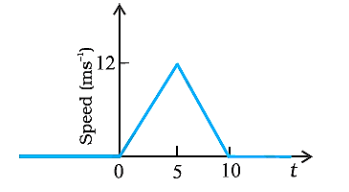What is the average speed of the particle over the intervals in (a) and (b)?
Solution:
(a) Distance travelled by the particle $=$ Area under the given graph
$=1 / 2 \times(10-0) \times(12-0)=60 \mathrm{~m}$
Average speed $=\frac{\text { Distance }}{\text { Time }}=\frac{60}{10}=6 \mathrm{~m} / \mathrm{s}$
(b) Let $s_{1}$ and $s_{2}$ be the distances covered by the particle between time
$t=2 \mathrm{~s}$ to $5 \mathrm{~s}$ and $\mathrm{t}=5 \mathrm{~s}$ to $6 \mathrm{~s}$ respectively
Total distance (s) covered by the particle in time $\mathrm{t}=2 \mathrm{~s}$ to $6 \mathrm{~s}$
$\mathrm{s}=\mathrm{s}_{1}+\mathrm{s}_{2} \ldots \ldots$ (i)
For distance $\mathrm{s}_{1}$ :
Let u' be the velocity of the particle after $2 \mathrm{~s}$ and a' be the acceleration of the particle in
$\mathrm{t}=0$ to $\mathrm{t}=5 \mathrm{~s}$
Since the particle undergoes uniform acceleration in the interval $\mathrm{t}=0$ to $\mathrm{t}=5 \mathrm{~s}$, from first equation of motion, acceleration can be obtained as:
$v=u+a t$
Where
$v=$ Final velocity of the particle
$12=0+\mathrm{a}^{\prime} \times 5$
$a^{\prime}=\frac{12}{5}=2.4 \mathrm{~m} / \mathrm{s}^{2}$
Again, from first equation of motion, we have
$\mathrm{v}=\mathrm{u}+\mathrm{at}$
$=0+2.4 \times 2=4.8 \mathrm{~m} / \mathrm{s}$
Distance travelled between $2 \mathrm{~s}$ and $5 \mathrm{~s}$ by the particle, i.e. in $3 \mathrm{~s}$
$s_{1}=u^{\prime} t+\frac{1}{2} a^{\prime} t^{2}$
$=4.8 \times 3+\frac{1}{2} \times 2.4 \times(3)^{2}$
$=25.2 \mathrm{~m} \ldots \ldots \ldots . .$ (ii)
For distance $\mathrm{S}_{2}:$
Let a" be the acceleration of the particle between time $\mathrm{t}=5 \mathrm{~s}$ and $\mathrm{t}=10 \mathrm{~s}$.
From the first equation of motion,’
$\mathrm{v}=\mathrm{u}+\mathrm{at}($ where $\mathrm{v}=0$ as the particle finally comes to rest $)$
$0=12+a^{\prime \prime} \times 5$
$a^{\prime \prime}=\frac{-12}{5}$
$=-2.4 \mathrm{~m} / \mathrm{s}^{2}$
Distance travelled by the particle in $1 \mathrm{~s}$ (i.e., between $\mathrm{t}=5 \mathrm{~s}$ and $\mathrm{t}=6 \mathrm{~s}$ )
$s_{2}=u t+\frac{1}{2} a^{\prime \prime} t^{2}$
$=12 \times \mathrm{a}+\frac{1}{2}(-2.4) \times(1)^{2}$
$=12-1.2=10.8 \mathrm{~m} \ldots \ldots \ldots$ (iii)
$=12-1.2=10.8 \mathrm{~m} \ldots \ldots \ldots$ (iii)
From equations (i), (ii), and (iii), we get
$\mathrm{s}=25.2+10.8=36 \mathrm{~m}$
$\therefore$ Average speed $=\frac{36}{4}=9 \mathrm{~m} / \mathrm{s}$

Question 28. The velocity-time graph of a particle in one-dimensional motion is shown in Figure.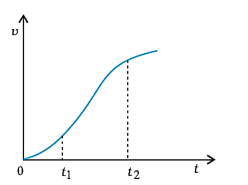Which of the following formulae are correct for describing the motion of the particle over the time-interval $t_{2}$ to $t_{1} ?$
(a) $x\left(t_{2}\right)=x\left(t_{1}\right)+v\left(t_{1}\right)\left(t_{2}-t_{1}\right)+\left(\frac{1}{2}\right) a\left(t_{2}-t_{1}\right)^{2}$
(b) $\quad v\left(t_{2}\right)=v\left(t_{1}\right)+a\left(t_{2}-t_{1}\right)$
(c) $v_{\text {Average }}=\frac{\left(x\left(t_{2}\right)-x\left(t_{1}\right)\right)}{t_{2}-t_{1}}$
(d) $a_{\text {Average }}=\frac{\left(v\left(t_{2}\right)-v\left(t_{1}\right)\right)}{t_{2}-t_{1}}$ (e) $x\left(t_{2}\right)=x\left(t_{1}\right)+v_{\text {Average }}\left(t_{2}-t_{1}\right)+\left(\frac{1}{2}\right) a_{\text {Average }}\left(t_{2}-t_{1}\right)^{2}$
(f) $x\left(t_{2}\right)-x\left(t_{1}\right)=$ area under the $v-t$ curve bounded by the t-axis and the dotted line shown.

Solution:
(c), (d) and, (f) describe the correct formulae of motion of a particle. The given graph has a non-uniform slope. Hence, the formulae given in (a), (b), and (e) cannot describe the motion of the particle. Only relations given in (c), (d), and (f) are correct equations of motion.’